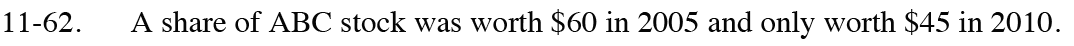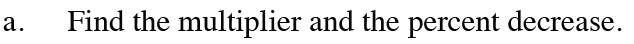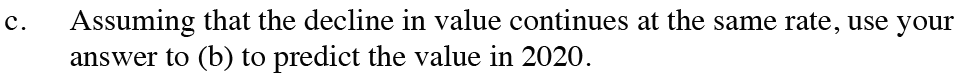Home > CC4 > Chapter 11 > Lesson 11.2.2 > Problem11-62

11-62.
1. A share of ABC stock was worth $60 in 2005 and only worth$45 in 2010. Homework Help ✎

1. Find the multiplier and the percent decrease.

2. Write an exponential function that models the value of the stock starting from 2005.

3. Assuming that the decline in value continues at the same rate, use your answer to (b) to predict the value in 2020.Create a table to help you find the multiplier.

Solve for m: 60 · m5 = 45

To find the percent decrease, divide the amount of the decrease by the starting value and convert to a percent.Use the general form of an exponential equation: y = a · mx, where m is the multiplier found in part (a), and a is the initial value.\$25.28